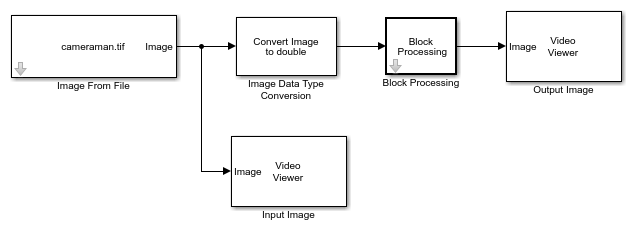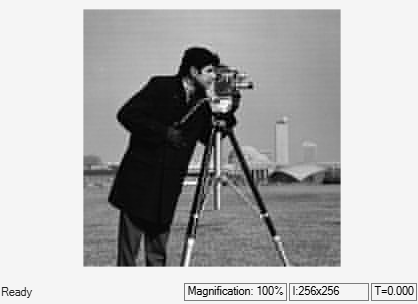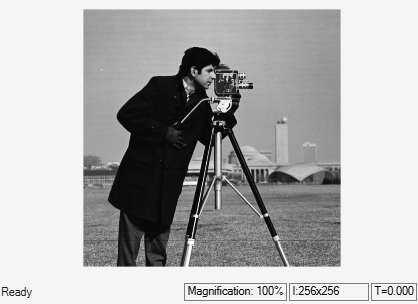# Compress Image Using 2-D DCT

This example shows how to compress an image using a 2-D discrete cosine transform (DCT). The example computes the 2-D DCT of 8-by-8 nonoverlapping blocks of the input image, discards (sets to zero) all but 10 of the 64 DCT coefficients in each block, and then reconstructs the image using the 2-D inverse discrete cosine transform (IDCT) of each block.

### Example Model

```modelname = 'ex_blk2ddct.slx'; open_system(modelname) ```The model reads an input image using the Image From File block, and converts the data type of the image to `double` using the Image Data Type Conversion block.

### Process Image Block-by-Block

The Block Processing block performs the operations defined in this subsystem on each block of the input image.The subsystem first computes the 2-D DCT of a block of the input image. The Product, Matrix Multiply (Simulink) block then multiplies the DCT coefficients by the elements of the mask, defined using the Constant (Simulink) block, to discard all but 10 of the 64 DCT coefficients. The 2-D IDCT block then reconstructs the processed image.

### Simulate and Display Results

Simulate the model. The Video Viewer blocks display the input and output images. Notice that, despite some loss of quality, the output image is clearly recognizable even after discarding nearly 85% of the DCT coefficients of the input image.

```sim(modelname); ```